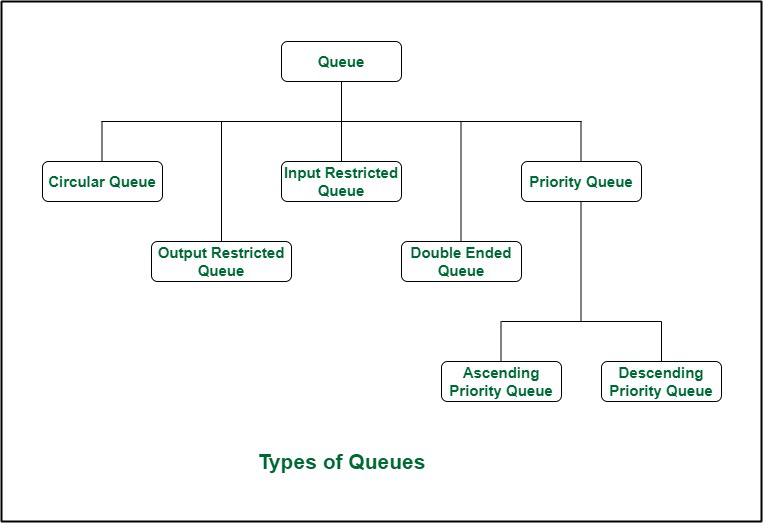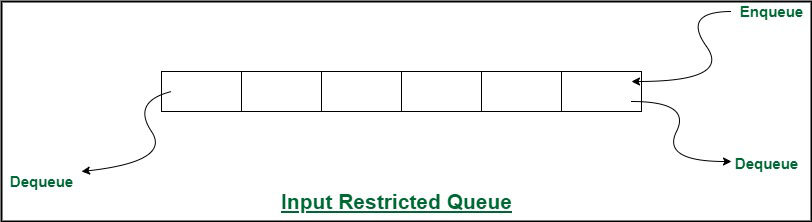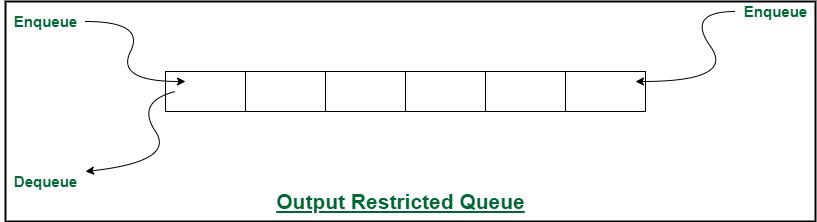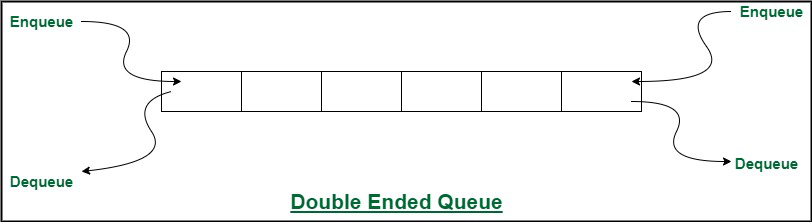Open in App
Not now

# Different Types of Queues and its Applications

• Difficulty Level : Medium
• Last Updated : 28 Mar, 2023

Introduction :

A Queue is a linear structure that follows a particular order in which the operations are performed. The order is First In First Out (FIFO). A good example of a queue is any queue of consumers for a resource where the consumer that came first is served first. In this article, the different types of queues are discussed.

Types of Queues:

There are five different types of queues that are used in different scenarios. They are:

1. Input Restricted Queue (this is a Simple Queue)
2. Output Restricted Queue (this is also a Simple Queue)
3. Circular Queue
4. Double Ended Queue (Deque)
5. Priority Queue
• Ascending Priority Queue
• Descending Priority QueueTypes of Queues

1. Circular Queue: Circular Queue is a linear data structure in which the operations are performed based on FIFO (First In First Out) principle and the last position is connected back to the first position to make a circle. It is also called ‘Ring Buffer’. This queue is primarily used in the following cases:

1. Memory Management: The unused memory locations in the case of ordinary queues can be utilized in circular queues.
2. Traffic system: In a computer-controlled traffic system, circular queues are used to switch on the traffic lights one by one repeatedly as per the time set.
3. CPU Scheduling: Operating systems often maintain a queue of processes that are ready to execute or that are waiting for a particular event to occur.

The time complexity for the circular Queue is O(1).

2. Input restricted Queue: In this type of Queue, the input can be taken from one side only(rear) and deletion of elements can be done from both sides(front and rear). This kind of Queue does not follow FIFO(first in first out).  This queue is used in cases where the consumption of the data needs to be in FIFO order but if there is a need to remove the recently inserted data for some reason and one such case can be irrelevant data, performance issue, etc.Input Restricted Queue

• Helps maintain stability and predictable performance of the system

• May lead to resource wastage if the restriction is set too low and items are frequently discarded
• May lead to waiting or blocking if the restriction is set too high and the queue is full, preventing new items from being added.

3. Output restricted Queue: In this type of Queue, the input can be taken from both sides(rear and front) and the deletion of the element can be done from only one side(front).  This queue is used in the case where the inputs have some priority order to be executed and the input can be placed even in the first place so that it is executed first.Output Restricted Queue

4. Double ended Queue: Double Ended Queue is also a Queue data structure in which the insertion and deletion operations are performed at both the ends (front and rear). That means, we can insert at both front and rear positions and can delete from both front and rear positions.  Since Deque supports both stack and queue operations, it can be used as both. The Deque data structure supports clockwise and anticlockwise rotations in O(1) time which can be useful in certain applications. Also, the problems where elements need to be removed and or added both ends can be efficiently solved using Deque.Double Ended Queue

5. Priority Queue: A priority queue is a special type of queue in which each element is associated with a priority and is served according to its priority. There are two types of Priority Queues. They are:

1. Ascending Priority Queue: Element can be inserted arbitrarily but only smallest element can be removed. For example, suppose there is an array having elements 4, 2, 8 in the same order. So, while inserting the elements, the insertion will be in the same sequence but while deleting, the order will be 2, 4, 8.
2. Descending priority Queue: Element can be inserted arbitrarily but only the largest element can be removed first from the given Queue. For example, suppose there is an array having elements 4, 2, 8 in the same order. So, while inserting the elements, the insertion will be in the same sequence but while deleting, the order will be 8, 4, 2.

The time complexity of the Priority Queue is O(logn).

Applications of a Queue:

The queue is used when things don’t have to be processed immediately, but have to be processed in First In First Out order like Breadth First Search. This property of Queue makes it also useful in the following kind of scenarios.

1. When a resource is shared among multiple consumers. Examples include CPU scheduling, Disk Scheduling.
2. When data is transferred asynchronously (data not necessarily received at the same rate as sent) between two processes. Examples include IO Buffers, pipes, file IO, etc.
3. Linear Queue: A linear queue is a type of queue where data elements are added to the end of the queue and removed from the front of the queue. Linear queues are used in applications where data elements need to be processed in the order in which they are received. Examples include printer queues and message queues.
4. Circular Queue: A circular queue is similar to a linear queue, but the end of the queue is connected to the front of the queue. This allows for efficient use of space in memory and can improve performance. Circular queues are used in applications where the data elements need to be processed in a circular fashion. Examples include CPU scheduling and memory management.
5. Priority Queue: A priority queue is a type of queue where each element is assigned a priority level. Elements with higher priority levels are processed before elements with lower priority levels. Priority queues are used in applications where certain tasks or data elements need to be processed with higher priority. Examples include operating system task scheduling and network packet scheduling.
6. Double-ended Queue: A double-ended queue, also known as a deque, is a type of queue where elements can be added or removed from either end of the queue. This allows for more flexibility in data processing and can be used in applications where elements need to be processed in multiple directions. Examples include job scheduling and searching algorithms.
7. Concurrent Queue: A concurrent queue is a type of queue that is designed to handle multiple threads accessing the queue simultaneously. Concurrent queues are used in multi-threaded applications where data needs to be shared between threads in a thread-safe manner. Examples include database transactions and web server requests.

Issues of Queue :

Some common issues that can arise when using queues:

1. Queue overflow: Queue overflow occurs when the queue reaches its maximum capacity and is unable to accept any more elements. This can cause data loss and can lead to application crashes.
2. Queue underflow: Queue underflow occurs when an attempt is made to remove an element from an empty queue. This can cause errors and application crashes.
3. Priority inversion: Priority inversion occurs in priority queues when a low-priority task holds a resource that a high-priority task needs. This can cause delays in processing and can impact system performance.
4. Deadlocks: Deadlocks occur when multiple threads or processes are waiting for each other to release resources, resulting in a situation where none of the threads can proceed. This can happen when using concurrent queues and can lead to system crashes.
5. Performance issues: Queue performance can be impacted by various factors, such as the size of the queue, the frequency of access, and the type of operations performed on the queue. Poor queue performance can lead to slower system performance and reduced user experience.
6. Synchronization issues: Synchronization issues can arise when multiple threads are accessing the same queue simultaneously. This can result in data corruption, race conditions, and other errors.
7. Memory management issues: Queues can use up significant amounts of memory, especially when processing large data sets. Memory leaks and other memory management issues can occur, leading to system crashes and other errors.

Reference :

Some references for further reading on queues:

1. “Data Structures and Algorithms in Java” by Robert Lafore – This book provides an in-depth explanation of different types of queues and their implementations in Java.
2. “Introduction to Algorithms” by Thomas H. Cormen et al. – This textbook covers the basic concepts of data structures and algorithms, including queues and their various applications.
3. “Concurrency in C# Cookbook” by Stephen Cleary – This book provides practical examples of how to use concurrent queues in C# programming.
4. “Queue (abstract data type)” on Wikipedia – This article provides an overview of queues and their properties, as well as examples of their applications.
5. “The Art of Computer Programming, Volume 1: Fundamental Algorithms” by Donald E. Knuth – This book includes a detailed analysis of different queue algorithms and their performance.
6. “Queues and the Producer-Consumer Problem” by Douglas C. Schmidt – This paper discusses how queues can be used to solve the producer-consumer problem in concurrent programming.

My Personal Notes arrow_drop_up
Related Articles